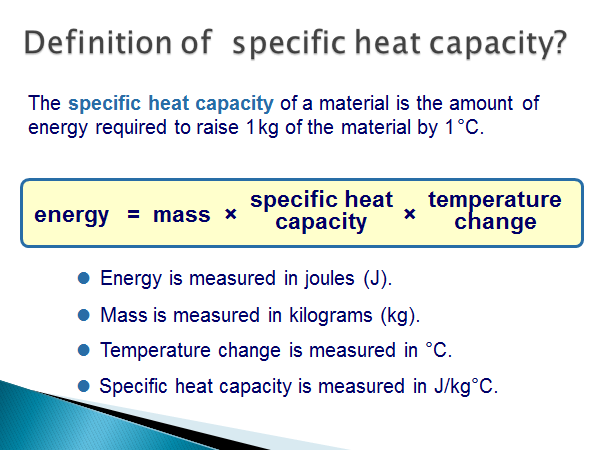# Energy Changes in Systems

## Define Specific Heat Capacity

Some objects heat up quicker than others. This depends on a property called specific heat capacity. The quicker an object heats up, the smaller its specific heat capacity. Specific Heat Capacity is the amount of energy required to raise the temperature of a 1kg substance by 1oC.

## Specific Heat Capacity Equation

The equation for specific heat capacity is as follows:This is also written as:## Using the Specific Heat Capacity Equation

Let’s do an example:

8.0kg of water is heater from 20°C to 40°C. The specific heat capacity of water is 4200J/kg°C. What is the energy supplied to the water?

First; write the equation:We can see from the question that m = 8kg and c = 4200J/kg°C.

The temperature change is 20°C, since 40°C - 20°C = 20°C.

We then substitute this into the equation:

E = 8 x 4200 x 20 = 672,000J or 672kJ

Specific heat capacity can appear in the five mark questions, mentioned in the previous lesson. This is because it has energy and mass in, which are easily substituted with different equations.

What is specific heat capacity?
Your answer should include: Amount / Energy / Required / Raise / Substance
Explanation: The amount of energy required to raise a 1kg substance by 1°C.
Calculate the energy needed to heat up 3.5kg of air from 80°C to 90°C? c = 1000J/kg°C